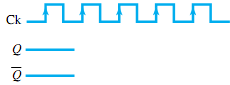## Complete the timing diagram for counter, Electrical Engineering

Assignment Help:

Q. When the J and K inputs of a JKFF are tied to logic 1, this device is known as a divide-by-2 counter. Complete the timing diagram shown in
Figure for this counter.#### Determine the power rating of compressor motor, An ice plant produces 12ton...

An ice plant produces 12tonn of ice per day at 0 0 C using water at 300C. The plant operates on reversed Carnot cycle between -15 0 C and 28 0 C. If the actual C.O.P. is 50% of the

#### Ac supply, its defination and mathematical expression if any

its defination and mathematical expression if any

#### Discuss the closed-loop behavior, Q. Discuss the closed-loop behavior? ...

Q. Discuss the closed-loop behavior? Consider an elementary feedback control system, as shown in Figure, with H = 1. The output variable c and the input e to the direct transmi

#### Basic characteristics and operation of mosfet, Q. Basic Characteristics and...

Q. Basic Characteristics and Operation of MOSFET ? In Figure the gate-to-source voltage is set to zero volts by the direct connection from one terminal to the other, and a volt

#### What is logic gates - microprocessor, What is Logic Gates? The Logic Gat...

What is Logic Gates? The Logic Gates are circuits made up of transistors, diodes, and resistors. The Logic gates process one or more input signals in a logical fashion. Dependin

#### P type material - semiconductor material, p type material: p type ma...

p type material: p type material is formed by doping aerial  crystal with impurity atoms having three valance electrons. The diffused impurities with three valence electrons

#### Explain norton''s theorem procedure, Norton's theorem procedure: 1.  Re...

Norton's theorem procedure: 1.  Remove R L from the circuit. Search I N by shorting links output terminal. 2.  Search R N by short-circuit voltage source or open-circuit

what is aphasor

#### Explain the neg instruction, Explain the NEG instruction. NEG: Two's c...

Explain the NEG instruction. NEG: Two's complement or arithmetic sign inversion (NEG). The NEG instruction two's complements a number that means that the arithmetic sign of a

#### Emf, discuss the factor affecting the emf induced in electric machines

discuss the factor affecting the emf induced in electric machines

### Write Your Message!#### Assured A++ Grade

Get guaranteed satisfaction & time on delivery in every assignment order you paid with us! We ensure premium quality solution document along with free turntin report!